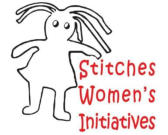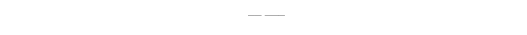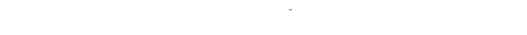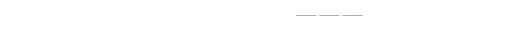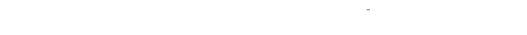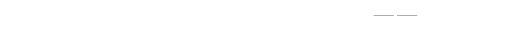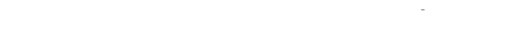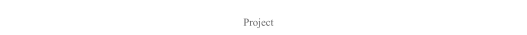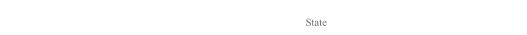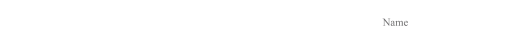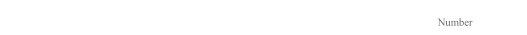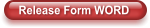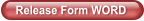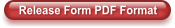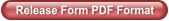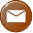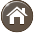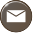STITCHES Release Form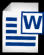STITCHES Womens Iniaitives 28056 Palomino Drive Warren, MI 48093  Phone: 586-873-8034
Releas e and   W aiv er Fo r m
Stitch es W o m en ’s  I n itiativ es
TH IS   IS I N F O R MAT I O N   IS   C O N FID E N TI A L
Eng l
i
sh/ E sp
an ol
N
am e
/
N
o m
b
r
e:
__ ______ _________ ______ ______ _ _________ ______ ______ _ _________ ______ _____
S t
r
e et  A
dd r
es s
:   ______ ___ _________ ______ ______ _ _________ ______ ______ _ _________ ______ ___
C
i t y
/
C
i ud
a
d:   ______ ____ ________
S
t at e
/
E
st a d
o
: _ ______ ____ _
Z i
p
/ Post a
l
C
od e_____ ____ _______
E
m
a
il / I n
t
e
r n
e t
:y
o
u
w a
nt
to be a dde
d
to
ou
r da ta ba se :________________ ____________________
I
g r an t ST I
T
C HE S W o m en
's
I n it
i
ati v e s   p er m i
s
s io n   to   u s e m
y
d o ll a n d   r elate d   in f o r m atio n   w it h o u t r estrictio n
,
e
x
ce p t w h er e
s p ec if ica
ll
y
s tated
,
in   an y   m e
d i
a f o r m .
I
u n d
e r
s
ta n d   th at t h is   d o ll m a y   b e
e
x h ib ited   an d   b r o ad
c
ast
l
o ca ll y
,
n at io n a
ll
y ,   an d   i n ter n ati o n all y
,
as ST
I
T
C
HE S sees f it
.
I   al
s o
u n d er s ta n d   th e d o ll m a
y
ap p ea r   in   m at
e
r ial u s ed   to   p r o m o te
,
p u b licize
,
o r   ex p lain   th e ST I T C HE S Do ll P r o j ec t
,   i
n clu d in
g
b u t n o t
l
i
m it
e
d   t
o
,
th e ST I T C HE
S w
e b
s
ite.
1 ex p r e
s
s l
y
r
elea s e ST I T
C
H
E
S W
o
m e n
'
s
I
n itiat
i
v es a n d   its   a g en t
s
o r   an
y
i n s tit u tio n   e
x h
ib it in g   t h
i
s   f r o m
a
n
y
clai m s   ar i
s
i n g   f r o m   s
u
c h
u s e o r   d i
s
tr ib u
t
i
o
n .   Als o
,
I
ag r ee   to   b e f u ll
y
r e
s
p o n
s
ib le
f o
r   m y   o w n   p ar ticip a
t
io n   i n   th i
s
p r o j ec t
,
an d   h o
l
d   ST I T C HE S
D
o
ll
P
r
o j ec t a
n d
i
ts   ag e
n
ts   h ar m les s
f o r
a
n
y
li
a b
il
it y
,
l
o s
s   o r   e
x
p
en s e ar is i n g
f r
o
m
t h e
u
s e
F
in all
y ,
1   ag r ee   to
w a
iv
e a
n
y
c o m p e n s at io n
f o
r   ta k in g   p ar t in   th
e
p r
o
d u ctio n
,
d is tr ib u ti
o
n
,
ex h ib i
t,
a n d   b r
o
a d
c a s
t o f   th is   d
o
ll a n d   an
y
r e
l
ated   p r
o
m
o
t
i
o n al o r   o r
g
a n
iz at
io n al m a ter ia
l.